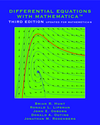Home
Project Description
Authors

Differential Equations
with Mathematica

Differential Equations
with Maple

Differential Equations
with Matlab

# Differential Equations with Mathematica, Third Edition

## Preface

© Copyright 2008 John Wiley and Sons, Inc.

Traditional introductory courses in ordinary differential equations (ODE) have concentrated on teaching a repertoire of techniques for finding formula solutions of various classes of differential equations. Typically, the result was rote application of formula techniques without a serious qualitative understanding of such fundamental aspects of the subject as stability, asymptotics, dependence on parameters, and numerical methods. These fundamental ideas are difficult to teach because they have a great deal of geometrical content and, especially in the case of numerical methods, involve a great deal of computation. Modern mathematical software systems, which are particularly effective for geometrical and numerical analysis, can help to overcome these difficulties. This book changes the emphasis in the traditional ODE course by using a mathematical software system to introduce numerical methods, geometric interpretation, symbolic computation, and qualitative analysis into the course in a basic way.

The mathematical software system we use is Mathematica™. (This book is also available in MATLAB and Maple versions.) We assume that the user has no prior experience with Mathematica. We include concise instructions for using Mathematica on any standard computer platform: Windows, Macintosh, or UNIX, including Linux. This book is not a comprehensive introduction or reference manual to either Mathematica or any of the computer platforms. Instead, it focuses on the specific features of Mathematica that are useful for analyzing differential equations. In this third edition we discuss some of the features of Mathematica 6 that make it even easier than it was previously to use Mathematica to produce integrated documents that incorporate text, mathematical calculations, and graphics.

This supplement can easily be used in conjunction with most ODE texts. It addresses the standard topics in ODE, but with a substantially different emphasis.

We had two basic goals in mind when we introduced this supplement into our course. First, we wanted to deepen students' understanding of differential equations by giving them a new tool, a mathematical software system, for analyzing differential equations. Second, we wanted to bring students to a level of expertise in the mathematical software system that would allow them to use it in other mathematics, engineering, or science courses. We believe that we have achieved these goals in our own classes. We hope this supplement will be useful to students and instructors on other campuses in achieving the same goals.

### Acknowledgement and Disclaimer

We are pleased to acknowledge support of our research by the National Science Foundation, which contributed over many years to the writing of this book. Our work on the third edition was partially supported by NSF Grants DMS-0616585, DMS-0611094, and DMS-0805003. Any opinions, findings, conclusions, or recommendations expressed in this material are those of the authors and do not necessarily reflect the views of the National Science Foundation.

Brian R. Hunt
Ronald L. Lipsman
John E. Osborn
Donald A. Outing
Jonathan M. Rosenberg

College Park, Maryland and West Point, New York
August, 2008